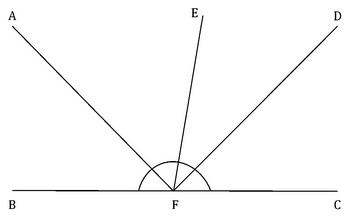Courses

# In the given figure, BC and EF are line segments intersecting at F. FD and AF are the angle bisectors of ∠EFC and ∠EFB respectively. Find the value ∠AFE + ∠DFE.a)45ob)60oc)90od)105oe)120oCorrect answer is option 'C'. Can you explain this answer? Related Test: Test: Line And Angles

## GMAT QuestionPrashant Kumar Jul 22, 2018
Let's CFE be x degree and EFB would be 180 degree- x degree . now DFE would be x/2 degree as FD is angle bisector similarly AFE would be 90-x/2 as AF is angle bisector too. Now DFE+ AFE = x/2+ 90-x/2= 90

This discussion on In the given figure, BC and EF are line segments intersecting at F. FD and AF are the angle bisectors of EFC and EFB respectively. Find the value AFE + DFE.a)45ob)60oc)90od)105oe)120oCorrect answer is option 'C'. Can you explain this answer? is done on EduRev Study Group by GMAT Students. The Questions and Answers of In the given figure, BC and EF are line segments intersecting at F. FD and AF are the angle bisectors of EFC and EFB respectively. Find the value AFE + DFE.a)45ob)60oc)90od)105oe)120oCorrect answer is option 'C'. Can you explain this answer? are solved by group of students and teacher of GMAT, which is also the largest student community of GMAT. If the answer is not available please wait for a while and a community member will probably answer this soon. You can study other questions, MCQs, videos and tests for GMAT on EduRev and even discuss your questions like In the given figure, BC and EF are line segments intersecting at F. FD and AF are the angle bisectors of EFC and EFB respectively. Find the value AFE + DFE.a)45ob)60oc)90od)105oe)120oCorrect answer is option 'C'. Can you explain this answer? over here on EduRev! Apart from being the largest GMAT community, EduRev has the largest solved Question bank for GMAT.

### Related Content

This discussion on In the given figure, BC and EF are line segments intersecting at F. FD and AF are the angle bisectors of EFC and EFB respectively. Find the value AFE + DFE.a)45ob)60oc)90od)105oe)120oCorrect answer is option 'C'. Can you explain this answer? is done on EduRev Study Group by GMAT Students. The Questions and Answers of In the given figure, BC and EF are line segments intersecting at F. FD and AF are the angle bisectors of EFC and EFB respectively. Find the value AFE + DFE.a)45ob)60oc)90od)105oe)120oCorrect answer is option 'C'. Can you explain this answer? are solved by group of students and teacher of GMAT, which is also the largest student community of GMAT. If the answer is not available please wait for a while and a community member will probably answer this soon. You can study other questions, MCQs, videos and tests for GMAT on EduRev and even discuss your questions like In the given figure, BC and EF are line segments intersecting at F. FD and AF are the angle bisectors of EFC and EFB respectively. Find the value AFE + DFE.a)45ob)60oc)90od)105oe)120oCorrect answer is option 'C'. Can you explain this answer? over here on EduRev! Apart from being the largest GMAT community, EduRev has the largest solved Question bank for GMAT.